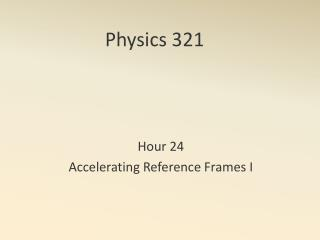DownloadDownload PresentationPhysics 321

# Physics 321

Download Presentation## Physics 321

- - - - - - - - - - - - - - - - - - - - - - - - - - - E N D - - - - - - - - - - - - - - - - - - - - - - - - - - -
##### Presentation Transcript

1. Physics 321 Hour 24 Accelerating Reference Frames I

2. Consider an accelerating train car

3. Glass of water in a car • Tides Examples

4. Euler’s Theorem • Any tiny rotation can be considered as a rotation about a fixed axis. • (Complex rotations can be taken to be a series of infinitesimal rotations.) • Tiny rotations commute. • (Larger rotations do not commute.) Two Angular Momentum Theorems

5. Proof

6. Proof

7. Any unit vector can be written as In general where S0 is the lab (inertial) frame and S’ is a frame rotating with angular velocity . Proof

8. Angular velocity vector (r.h.r.) • Let be a unit vector fixed in a body • In particular • In general Angular Velocity

9. Begin Handout